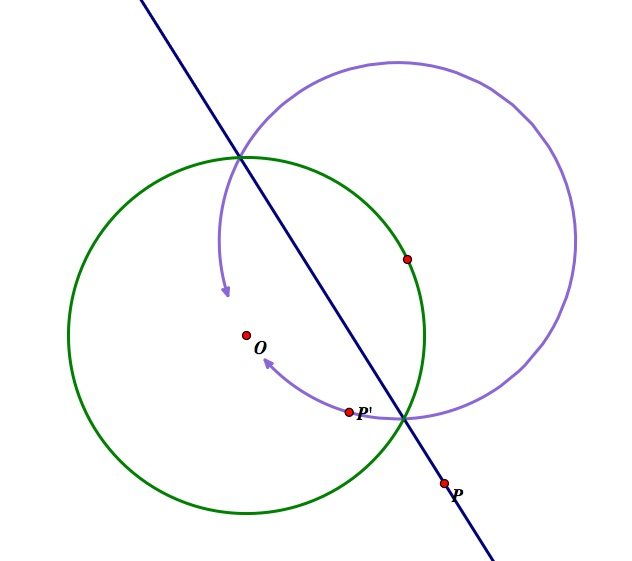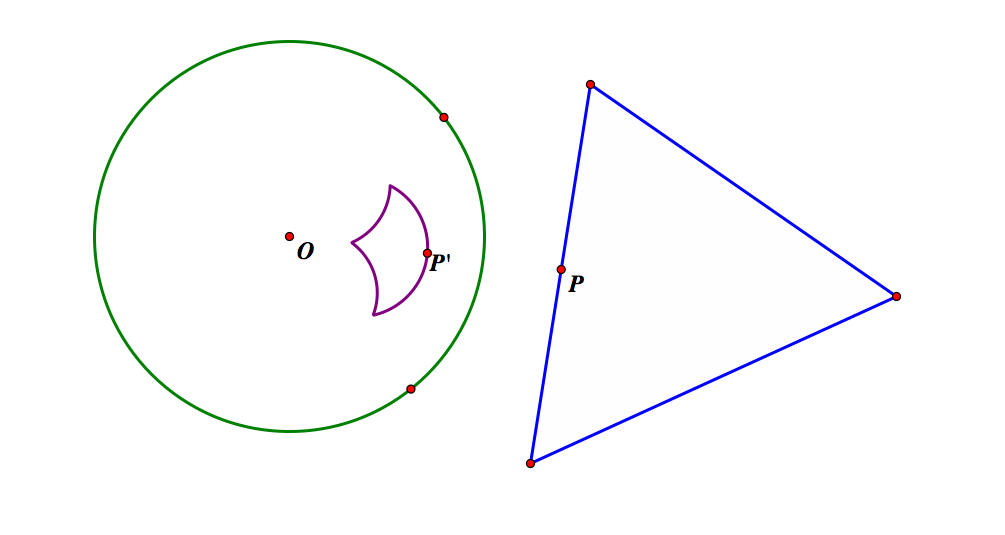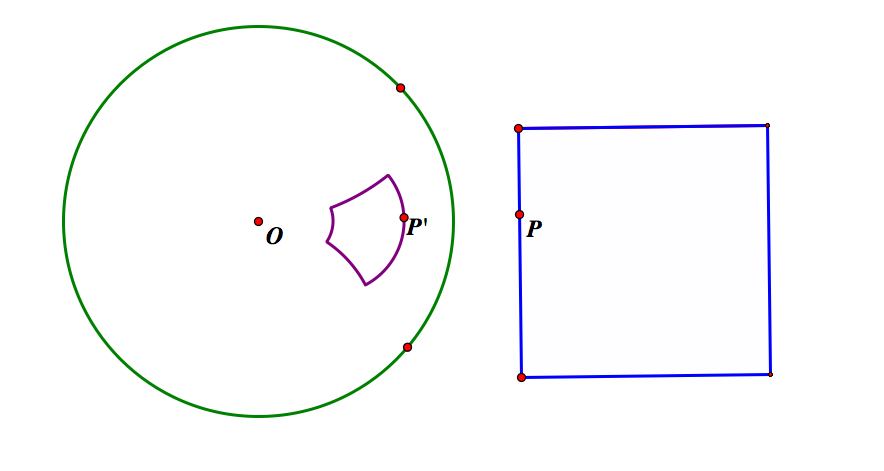Department of Mathematics Education
EMAT 4000/6000.  J.Wilson, Instructor

Excursion Through Inversion

By Lexi Stear, Mimi Tsui, and Kendyl Wade

Introduction:

“An inversion in a circle, informally, is a transformation of a plane that flips the circle inside-out. That is, points outside the circle get mapped to points inside the circle and points inside the circle get mapped outside the circle.” (Kozai and Libeskind)

Within inversive geometry, all the points inside a circle map to the points outside the circle and all the points outside the circle map to points inside the circle. The circle of inversion will be referred to as C and the center of C will be consistently labeled O.  The inverse of a point P will be labeled as P’, and r refers to the radius of the circle of inversion.  The point P’ is determined such that

OP*OP’ =.

And when r = 1,

OP*OP’ = 1

OP = 1/(OP’) =.

So when P is a point on the circle of inversion, P’ is the same point. The farther P gets from the circle of inversion, the closer P’ gets to the center O. Thus, a point P at infinity maps to the center O.

For an interactive visual, open this GSP file.

Now let’s observe what happens when we take the inverse of circles and lines with respect to a circle of inversion C:Here we have taken the inverse of a line that passes through the center of C.

Note that the inverse of the line is itself.Now we have created a line that does not pass through the center O and is outside of C. The inverse of this line is a circle inside C that passes through O. We know a line goes on to infinity in two opposing directions, and since infinity maps to the center of inversion, it makes sense that the inverse passes through O.

Similarly, if you were to construct a line that passes through C but does not pass through the center, the inverse would be a circle outside of C.Here, we constructed a line that was tangent to the circle of inversion.

The inverse of this line is a circle that passes through O and is also tangent to C at the same point that the line is tangent.If P traces a line that intersects the circle of inversion, P’ will trace a circle that intersects the circle of inversion at the same points as the line.Here we have constructed a circle outside of C and taken it’s inverse. The result was a smaller circle inside of C. Also, although you cannot tell in the image, the circle has changed orientation. If you were to drag P in a clockwise direction around the circle, P’ would move counter-clockwise on the inverse.

Similarly, if we were to construct a circle inside C (that does not pass through O), the inverse would be a circle outside of C.Here, we have constructed a circle that is orthogonal to the circle of inversion. Taking the inverse of this circle results in itself. However, if P moves clockwise, P’ moves counter-clockwise.

(Note: A circle that is orthogonal to another circle means that they intersect at 90 degree angles.)

Angles are preserved under inversion. If two curves intersect at angle a, their inverse will also intersect at angle a, but in a reversed direction of sweeping.Here we have four circles tangent at and passing though O and a fifth circle passing through O, but not tangent to the other circles. If we take the inverse of all these circles, we get five lines: four that are parallel and one that is intersecting the parallel lines (and is thus a transversal). Under inversion, the angles made by the parallel lines and the transversal have the same measurement as the angles made by the intersection of the original circles.

Proof of angle preservation (lemma 9.3, page 70): http://www.ms.uky.edu/~droyster/courses/spring04/classnotes/Chapter%2009.pdf

The circles of the chessboard inversion intersect at right angles. All the circles are centered on either the x-axis or y-axis, and they would all share a common point at the origin, if the circles were complete. The flower shaped hole at the origin is originally the boundary of the chessboard.

For the following two images, the green circle is the circle of inversion. The blue is the path of P and the purple is the path of P’:Here we have taken the inverse of a triangle with respect to a circle outside of the triangle.Here we have taken the inverse of a square with respect to a circle outside of the square.

For a small exploration of inversion with triangles, open this GSP file.

Now let's do some exploring with taking the inverse of conics...When the inversion circle is centered at the focal point, the inverse of a parabola (brown) is a cardioid (red).When the circle of inversion is centered at the center of a hyperbola (brown), the inverse is a lemniscate (aqua).When the circle of inversion is centered at one of the foci, the resulting inverse is a limacon (aqua).

Applications of Inversion:

There are a variety of applications associated with Inversion in geometry.

The main applications include:

• Apollonius' Problem
• Steiner Chain

Other uses include:
• Pappus Chain
• Magnetism
• Poincaré Disk

Apollonius' Problem:

Given three circles, which are non-intersecting and of different radii length,find a fourth circle that is tangent to all three circles.To see how Apollonius' problem can be solved with inversion, open this GSP file.

Steiner Chain:

A Steiner chain is a set of circles where one circle is inside of another, then between the two circles there is a chain of n circles all tangent to each other.In order to create this chain, we can start with two circles that are concentric and create a chain of congruent, tangent circles between them. Then, by choosing a particular spot for the circle of inversion, we can create the above image.History of Inversion:

Inversion dates back to more than 2000 years ago. Apollonius of Perga (225 B.C. - 190 B.C.) investigated one particular family of curves (the Apollonius curve), which is significant in inversive geometry. He defined the Apolloniancurve C sub k(A,B) to be the locus of points P such that PA = kPB, where A and B are points in the Euclidean plane, and k is a positive constant. He gave the following theorem:

1) C sub k(A,B) is a line if k = 1 and a circle for all other positive k.

2) if C sub k(A,B) is a circle with center O and radius r, then

a) O,A,B are collinear with A and B on the same side of O.

b) OA.OB =c) If A,B, and the given circle satisfy a) and b) then the circle is an Apollonian curve.

He also proposed the problem to construct a circle that is tangent to three circles. This problem can be solved using inversion. Dandelin solved this problem using inversion in a memoir in 1825.

In 330 A.D. Pappus of Alexandria (290-350)  was the first to mention that this transformation carried a line or circle into a line or circle. However, a more in depth study of inversion did not happen until the 19th century.

Steiner (1769-1863) wrote many papers on the subject of inversion, but he never formally published them before he died. He explicitly states that the inverse of a parabola is a closed curve passing through the center of inversion C; that the inverse of an hyperbola always goes through C and the asymptotes transform into two asymptotic circles. However, he does not provide any proof as to how he arrived at these conclusions.

The quasi-transformation, “Given a circle w with center O and radius k and a point P different from O, we define the inverse of P to be the point P’, on the ray OP, whose distance from O satisfies the equationOPOP'=k2” was invented by L. J. Magnus in 1831 (Coxeter and Greitzer, p. 108). Ludwig Immanuel Magnus (1790-1861) was a German Jewish mathematician.

Bellavitis (1803-1880) discusses inversion nearly completely in a paper in 1836, but it is clear he does acknowledge that he is not the first to study the material.

In Education:

“Dynamic geometry environments provide students with experimental and modeling tools that allow them to investigate geometric phenomena in much the same way as computer algebra systems allow them to experiment with algebraic phenomena.” -Common Core Standards for Mathematics

Connections:

Since inversion is not mentioned in the standards explicitly, we have to take bits and pieces from the Geometry section and expand on them to include inversion.

Teaching inversion is useful for challenging students and motivating them to work on more in-depth and difficult problems.

Make Geometric Constructions:

12. Make formal geometric constructions with a variety of tools and methods.

→Using tools such as GSP allows students to explore Inversion Geometry by making formal constructions. After making a specific tool that inverts, it is easier to explore properties of inversion with different shapes, centers of inversion, etc.

Example: Constructing P’ (inside and outside the circle of inversion).

13. Construct an equilateral triangle, a square, and a regular hexagon inscribed in a circle.

→Use GSP to make these constructions, and then invert them using the circumcircle as the circle of inversion.

Understand and Apply Theorems about Circles:

3. Construct the inscribed and circumscribed circles of a triangle, and prove properties of angles for a quadrilateral inscribed in a circle.

→After constructing and inverting in GSP, have students make observations, especially about what happens to the angles in the inversion image.

References:

What is Mathematics? By Richard Courant and Herbert Robbins. Copyright, 1941, by Richard Courant.

Geometry Revisited by H.S.M. Coxeter and S.L. Greitzer. Copyright, 1967, by Yale University.

Common Core State Standards for Mathematics

The Origins of the Geometric Principle of Inversion by Boyd C. Patterson. Published by the University of Chicago Press, 1933.

Circle Inversions and Applications to Euclidean Geometry by Kenji Kozai and Shlomo Libeskind. 2009.

http://www.ma.utexas.edu/users/gilbert/M333L/chp5vers4.pdf

http://www.sanjosemathcircle.org/handouts/2010-2011/20110323_inversion.pdf

http://www.cut-the-knot.org/ctk/Circle.shtml

Inverses of curves: http://www-groups.dcs.st-and.ac.uk/~history/Curves/Curves.html

For a brief presentation on Inversion, go here.

Return